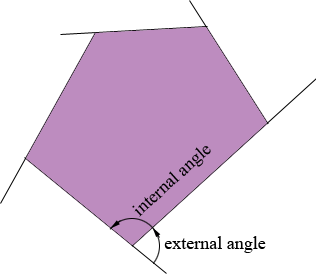SEARCH HOMEMath Central Quandaries & QueriesQuestion from victoria, a student: The sum of the measure of two angles of a pentagon is 240. If the remaining angles are congruent, what is the measure of each angle?Hi Victoria,This is probably easiest if you consider the sum of the measures of the 5 exterior angles. The sum of the measures of the 5 exterior angles and the 5 interior angles is 5 × 180o = 900o. Subtract the sum of the measures of the 5 exterior angles from 900o to find the sum of the measures of the 5 interior angles. You know that the sum of the measure of two of the interior angles is 240o. How much is left to distribute among the 3 remaining angles?

PennyMath Central is supported by the University of Regina and The Pacific Institute for the Mathematical Sciences.